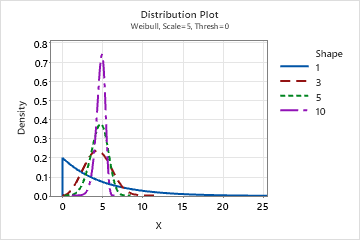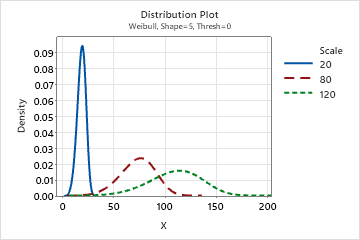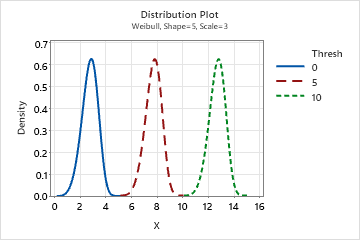# Weibull distribution

The Weibull distribution is a versatile distribution that can be used to model a wide range of applications in engineering, medical research, quality control, finance, and climatology. For example, the distribution is frequently used with reliability analyses to model time-to-failure data. The Weibull distribution is also used to model skewed process data in capability analysis.

The Weibull distribution is described by the shape, scale, and threshold parameters, and is also known as the 3-parameter Weibull distribution. The case when the threshold parameter is zero is called the 2-parameter Weibull distribution. The 2-parameter Weibull distribution is defined only for positive variables. A 3-parameter Weibull distribution can work with zeros and negative data, but all data for a 2-parameter Weibull distribution must be greater than zero.

Depending on the values of its parameters, the Weibull distribution can take various forms.

Effect of the shape parameter
The shape parameter describes how your data are distributed. A shape of 3 approximates a normal curve. A low value for shape, say 1, gives a right-skewed curve. A high value for shape, say 10, gives a left-skewed curve.Effect of the scale parameter
The scale, or characteristic life, is the 63.2 percentile of the data. The scale defines the position of the Weibull curve relative to the threshold, which is analogous to the way the mean defines the position of a normal curve. A scale of 20, for example, indicates that 63.2% of the equipment will fail in the first 20 hours after the threshold time.Effect of the threshold parameter
The threshold parameter describes the shift of the distribution away from 0. A negative threshold shifts the distribution to the left, and a positive threshold shifts the distribution to the right. All data must be greater than the threshold. The 2-parameter Weibull distribution is the same as the 3-parameter Weibull with a threshold of 0. For example, the 3-parameter Weibull (3,100,50) has the same shape and spread as the 2-parameter Weibull (3,100), but is shifted 50 units to the right.